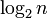# Unitriangular matrix group:UT(3,9)

View a complete list of particular groups (this is a very huge list!)[SHOW MORE]

## Definition

This group is the unitriangular matrix group of degree three (i.e., the group of$3 \times 3$ upper-triangular matrices with$1$s on the diagonal) over the field of nine elements. It is isomorphic to the 3-Sylow subgroup of general linear group:GL(3,9), special linear group:SL(3,9), projective general linear group:PGL(3,9), projective special linear group:PSL(3,9).

## Arithmetic functions

Want to compare and contrast arithmetic function values with other groups of the same order? Check out groups of order 729#Arithmetic functions

### Basic arithmetic functions

Function Value Similar groups Explanation
underlying prime of p-group 3
order (number of elements, equivalently, cardinality or size of underlying set) 729 groups with same order As$UT(n,q), q = 9, n = 3$:$q^{n(n-1)/2} = 9^{3(2)/2} = 729$
prime-base logarithm of order 6 groups with same prime-base logarithm of order
max-length of a group 6 max-length of a group equals prime-base logarithm of order for group of prime power order
chief length 6 chief length equals prime-base logarithm of order for group of prime power order
composition length 6 composition length equals prime-base logarithm of order for group of prime power order
exponent of a group 3 groups with same order and exponent of a group | groups with same exponent of a group
prime-base logarithm of exponent 1 groups with same order and prime-base logarithm of exponent | groups with same prime-base logarithm of order and prime-base logarithm of exponent | groups with same prime-base logarithm of exponent
Frattini length 2 groups with same order and Frattini length | groups with same prime-base logarithm of order and Frattini length | groups with same Frattini length
nilpotency class 2 groups with same order and nilpotency class | groups with same prime-base logarithm of order and nilpotency class | groups with same nilpotency class As$UT(n,q), n = 3$: nilpotency class is$n - 1 = 3 - 1 = 2$
derived length 2 groups with same order and derived length | groups with same prime-base logarithm of order and derived length | groups with same derived length As$UT(n,q), n = 3$: derived length is the smallest integer greater than or equal to$\log_2n$.
minimum size of generating set 4 groups with same order and minimum size of generating set | groups with same prime-base logarithm of order and minimum size of generating set | groups with same minimum size of generating set As$UT(n,q), q = p^r, n = 3, r = 2$:$r(n - 1) = 2(3 - 1) = 2(2) = 4$
subgroup rank of a group 4 groups with same order and subgroup rank of a group | groups with same prime-base logarithm of order and subgroup rank of a group | groups with same subgroup rank of a group
rank of a p-group 4 groups with same order and rank of a p-group | groups with same prime-base logarithm of order and rank of a p-group | groups with same rank of a p-group
normal rank of a p-group 4 groups with same order and normal rank of a p-group | groups with same prime-base logarithm of order and normal rank of a p-group | groups with same normal rank of a p-group
characteristic rank of a p-group 2 groups with same order and characteristic rank of a p-group | groups with same prime-base logarithm of order and characteristic rank of a p-group | groups with same characteristic rank of a p-group

## GAP implementation

### Group ID

This finite group has order 729 and has ID 469 among the groups of order 729 in GAP's SmallGroup library. For context, there are groups of order 729. It can thus be defined using GAP's SmallGroup function as:

SmallGroup(729,469)

For instance, we can use the following assignment in GAP to create the group and name it$G$:

gap> G := SmallGroup(729,469);

Conversely, to check whether a given group$G$ is in fact the group we want, we can use GAP's IdGroup function:

IdGroup(G) = [729,469]

or just do:

IdGroup(G)

to have GAP output the group ID, that we can then compare to what we want.

### Other descriptions

Description Functions used
SylowSubgroup(SL(3,9),3) SylowSubgroup, SL
SylowSubgroup(GL(3,9),3) SylowSubgroup, GL